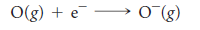×
Get Full Access to Chemistry: The Central Science - 12 Edition - Chapter 8 - Problem 103ie
Get Full Access to Chemistry: The Central Science - 12 Edition - Chapter 8 - Problem 103ie

×

# The electron affinity of oxygen is , corresponding to theISBN: 9780321696724 27

## Solution for problem 103IE Chapter 8

Chemistry: The Central Science | 12th Edition

• Textbook Solutions
• 2901 Step-by-step solutions solved by professors and subject experts
• Get 24/7 help from StudySoup virtual teaching assistantsChemistry: The Central Science | 12th Edition

4 5 1 320 Reviews
17
5
Problem 103IE

The electron affinity of oxygen is -141 kj/mol, corresponding to the reactionThe lattice energy of K2O(s) is 2238 kj/mol. Use these data along with data in Appendix C and Figure 7.9 to calculate the “second electron affinity” of oxygen, corresponding to the reactionStep-by-Step Solution:
Step 1 of 3

Plant Physiology Exam 4 Study Guide Accumulation and partitioning of photosynthesis­ starch and sucrose ­fate of triose phosphates produced as a result of the Calvin­Benson cycle ­remain in chloroplast or exported to cytosol ­in either site they are converted to hexose (6C) sugars ­hexose sugars are used for either starch (chloroplast) or sucrose (cytosol) synthesis (or respiration or growth) Starch synthesis ­begins with hexose phosphate pools produced by the PCR (CB) cycle (regen phase) ­Fructose­6­phosphate is converted to glucose­1 phosphate isomerase…. ­Synthesized into the stroma of plastids in 2 principle structures ­amylose (linear glucose polymer with alpha 1, 4 linkages) and amylopecti

Step 2 of 3

Step 3 of 3

##### ISBN: 9780321696724

Chemistry: The Central Science was written by and is associated to the ISBN: 9780321696724. This textbook survival guide was created for the textbook: Chemistry: The Central Science, edition: 12. Since the solution to 103IE from 8 chapter was answered, more than 312 students have viewed the full step-by-step answer. The answer to “?The electron affinity of oxygen is -141 kj/mol, corresponding to the reaction The lattice energy of K2O(s) is 2238 kj/mol. Use these data along with data in Appendix C and Figure 7.9 to calculate the “second electron affinity” of oxygen, corresponding to the reaction” is broken down into a number of easy to follow steps, and 44 words. This full solution covers the following key subjects: affinity, reaction, oxygen, electron, corresponding. This expansive textbook survival guide covers 49 chapters, and 5471 solutions. The full step-by-step solution to problem: 103IE from chapter: 8 was answered by , our top Chemistry solution expert on 04/03/17, 07:58AM.

Unlock Textbook Solution